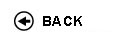Politecnico di Torino
Academic Year 2009/10
02BQZDR, 02BQZAX, 02BQZAY, 02BQZDS
Mathematical methods for engineering II
1st degree and Bachelor-level of the Bologna process in Mechanical Engineering - Vercelli
1st degree and Bachelor-level of the Bologna process in Civil Engineering - Vercelli
Master of science-level of the Bologna process in Civil Engineering - Vercelli
Espandi...
 Teacher Status SSD Les Ex Lab Tut Years teaching Gamba Andrea Antonio A2 MAT/07 30 30 0 0 9
 SSD CFU Activities Area context MAT/05 6 D - A scelta dello studente A scelta dello studente
 Objectives of the course The course provides basic knowledge about ordinary and partial differential equations and their applications. Expected skills Being able to integrate ordinary differential equations (ODE) with forcing terms. Computing eigenfunctions and eigenvalues of simple linear differential operators. Reducing a partial differential equation (PDE) to its canonic form. Solving the wave equation using D'Alembert's method. Deriving the equation for small vibrations of a string or membrane, the heat equation, the equations of momentum and energy balance for a perfect fluid. Solving simple linear PDE (wave equation, heat equation) with a variety of forcing terms, boundary conditions, and initial conditions, both in the transitory and stationary regime. Prerequisites Calculus I, II, Linear algebra, Mathematical methods I Syllabus Ordinary differential equations (ODE) with costant coefficients with a forcing term. Damped spring-mass system. Resonance. Principle of superposition. Conservative ODE's. Eigenvalues and eigenfunctions of linear differential operators. Linear partial differential equations (PDE). Variable change in a PDE: geometric meaning, transformation of derivative terms. Equations of characteristic curves. PDE classification: elliptic, parabolic, hyperbolic PDE's. Main examples: wave equation, heat equation, Laplace equation. Small, transverse vibrations of a string. Small, longitudinal vibrations of a bar. Divergence theorem. Small, transverse vibrations of a membrane. Hydrodynamic equations for perfect fluids: Euler equations, continuity equation, equation of state. Linearization of hydrodynamic equations close to equilibrium, wave equation. Boundary conditions for PDE's. Theorem of uniqueness for the equation of small vibrations. Small vibrations of an infinite string, D'Alembert's method. Small vibrations of a finite string, method of images. Sturm-Liouville problems: eigenvalues, eigenfunctions, distribution of energy on normal modes. Laboratories and/or exercises Exercises about the arguments of the course are discussed and solved in the class. Bibliography Haberman, 'Elementary applied partial differential equations with Fourier series and boundary value problems ', Prentice Hall , 1987 Tikhonov, Samarskii, 'Equations of Mathematical Physics', Dover, 1990 Kreyszig, 'Advanced Engineering Mathematics', Wiley, 1998 Revisions / Exam The exam consists of written exercises and questions about the arguments discussed in the course. Programma definitivo per l'A.A.2009/10© Politecnico di Torino
Corso Duca degli Abruzzi, 24 - 10129 Torino, ITALY